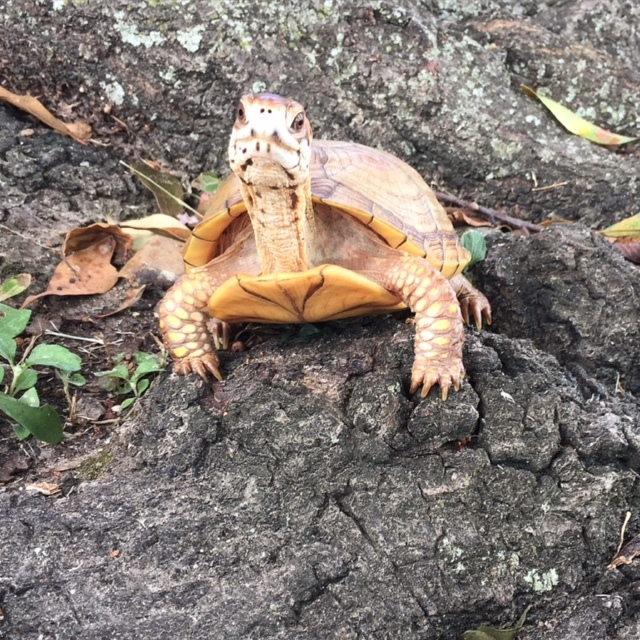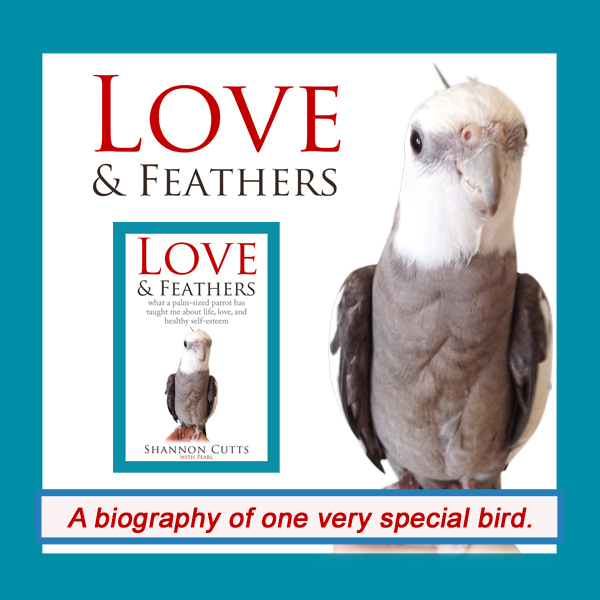# May 2018

 table div table+table+table div table{width:100%;padding:0}table div table+table+table div table img{width:96.23%;padding:0;float:none}table div table+table+table div table td{width:100%;padding:0 1.88% 18px}/* styles *//* styles */ Receiving this email as a forward? Sign up to keep following our little flock's adventures!
 table div table+table+table+table+table+table div table{width:100%;padding:0}table div table+table+table+table+table+table div table img{width:96.23%;padding:0;float:none}table div table+table+table+table+table+table div table td{width:100%;padding:0 1.88% 18px}/* styles */## Your Body Loves You UnconditionallyThis is one of my favorite pics of Bruce! He is so at one with his body - they are partners. His confidence and connection just shines through and he really mentors me by his example.

"Your body loves you unconditionally." I will never forget reading those lines for the first time just a few months ago.

I had signed up for a course about healing through the natural properties in foods. Blueberries, bananas, spinach, kale....these were my new study buddies.

I was so struck by the kindness in the fruits and vegetables and grains and spices I was learning about. They each seemed chock-full of exactly the sorts of nutrients my body needs the most.

The more I learned, the more I started to think this isn't any accident. In reflecting back on my Native American ancestors, I thought how intuitive feeding the body must have seemed to them.

Certain plants grew in certain seasons, and they ate these plants as naturally as the Earth's seasons came and went. Since it took some time to grow (or hunt) the food they needed, they also had plenty of time to learn about each food and notice how each was a healer in its own way.

Same with the fascinating world of herbs - a tiny green plant could pack quite a punch! More importantly, no one took its powers for granted or discounted its worth just because it was alive and had roots and was readily available for all who needed it.

Their bodies had to be patient, too. No one had taught them that impatience was an option. If you were hungry, it was time to go grow something, or hunt something, or pick something. Until you did, you would just stay hungry.

Of course I am just guessing about all this - I never lived as an Indian, didn't even meet my great great grandmother who walked (and died) on the Trail of Tears.

And yet, I think there is something of her still inside of me, because when given the chance to learn about my favorite fruits and veggies, I find I am an eager student. And I also have plenty of motivation to study, because to date modern medical knowledge hasn't given me the relief I need. So I am counting on these simple green things to help my chronically aching body heal.

On that note, the food and healing course I am taking didn't actually start out talking about blueberries. It started out by talking about bodies. Bodies are with us every step of the way throughout our life. My body is present for every second of my waking life and every moment of sleep.

When I ask my body to go somewhere or do something, it does it, no matter how much it hurts it to comply. Even if it is still tired, it gets up out of bed when I ask it to. It allows itself to be poked and prodded and tested and examined on my behalf.

My body eats what I give it, no matter whether I make healthy or unhealthy eating choices. And it tries its utmost to make the best of things, given the state of my emotions, heart and spirit on any given day.

If there was ever a mentor who truly shows me unconditional love, it would be my own body. The more I ponder this, the more I feel sad when I fall short again and again in loving it back with the same level of unquestioning devotion.

But then I ponder what writer and doctor Rachel Naomi Remen has to say about the purpose of life, which is "to grow in wisdom and learn to love better."

My body loves me unconditionally.

Now that I know this, feel in my heart and soul that this is true, I have the opportunity to grow in wisdom and learn to love it so much better.

With great respect and love,

Shannon

 table div table+table+table+table+table+table+table+table+table div table{width:100%;padding:0}table div table+table+table+table+table+table+table+table+table div table img{width:96.23%;padding:0;float:none}table div table+table+table+table+table+table+table+table+table div table td{width:100%;padding:0 1.88% 18px}/* styles */## How I try to love my body better:

▪ By making time each morning to stretch and do some yoga.
▪ By staying strong when it craves foods I know will hurt it.
▪ By working with my mind and emotions to help them support my body better as well.
▪ By meditating on all the things I'm grateful to my body for giving me.
▪ By visualizing a healthy, happy body that feels great.
 ▪ By making time each morning to stretch and do some yoga.
 ▪ By staying strong when it craves foods I know will hurt it.
 ▪ By working with my mind and emotions to help them support my body better as well.
 ▪ By meditating on all the things I'm grateful to my body for giving me.
 ▪ By visualizing a healthy, happy body that feels great.
 table div table+table+table+table+table+table+table+table+table+table+table div table{width:100%;padding:0}table div table+table+table+table+table+table+table+table+table+table+table div table img{width:96.23%;padding:0;float:none}table div table+table+table+table+table+table+table+table+table+table+table div table td{width:100%;padding:0 1.88% 18px}/* styles */# Get your copy of Love & Feathers the book!Click on the image of Pearl to order your signed copy!Pearl will even beak-o-graph it for you!
 table div table+table+table+table+table+table+table+table+table+table+table+table+table+table+table+table div table{width:100%;padding:0}table div table+table+table+table+table+table+table+table+table+table+table+table+table+table+table+table div table img{width:96.23%;padding:0;float:none}table div table+table+table+table+table+table+table+table+table+table+table+table+table+table+table+table div table td{width:100%;padding:0 1.88% 18px}/* styles */# We want what Malti's having!!

 table.module-17{width:75.47%;padding:0}table div table+table+table+table+table+table+table+table+table+table+table+table+table+table+table+table+table+table div table{width:75.47%;float:none;margin-left:auto;margin-right:auto;padding:0}table div table+table+table+table+table+table+table+table+table+table+table+table+table+table+table+table+table+table div table a{border:0 none;text-decoration:none}table div table+table+table+table+table+table+table+table+table+table+table+table+table+table+table+table+table+table div table img{width:100%!important;border:0 none;text-decoration:none}table div table+table+table+table+table+table+table+table+table+table+table+table+table+table+table+table+table+table div table td{width:100%;padding:0}/* styles */
 table div table+table+table+table+table+table+table+table+table+table+table+table+table+table+table+table+table+table+table+table div table{width:100%;padding:0}table div table+table+table+table+table+table+table+table+table+table+table+table+table+table+table+table+table+table+table+table div table img{width:96.23%;padding:0;float:none}table div table+table+table+table+table+table+table+table+table+table+table+table+table+table+table+table+table+table+table+table div table td{width:100%;padding:0 1.88% 18px}/* styles */# Bruce's Texas box turtle roots really show!

 table.module-21{width:75.47%;padding:0}table div table+table+table+table+table+table+table+table+table+table+table+table+table+table+table+table+table+table+table+table+table+table div table{width:75.47%;float:none;margin-left:auto;margin-right:auto;padding:0}table div table+table+table+table+table+table+table+table+table+table+table+table+table+table+table+table+table+table+table+table+table+table div table a{border:0 none;text-decoration:none}table div table+table+table+table+table+table+table+table+table+table+table+table+table+table+table+table+table+table+table+table+table+table div table img{width:100%!important;border:0 none;text-decoration:none}table div table+table+table+table+table+table+table+table+table+table+table+table+table+table+table+table+table+table+table+table+table+table div table td{width:100%;padding:0}/* styles */
 table div table+table+table+table+table+table+table+table+table+table+table+table+table+table+table+table+table+table+table+table+table+table+table+table div table{width:100%;padding:0}table div table+table+table+table+table+table+table+table+table+table+table+table+table+table+table+table+table+table+table+table+table+table+table+table div table img{width:96.23%;padding:0;float:none}table div table+table+table+table+table+table+table+table+table+table+table+table+table+table+table+table+table+table+table+table+table+table+table+table div table td{width:100%;padding:0 1.88% 18px}/* styles */## Meet Pearl, Malti, Bruce & Me

 table div table+table+table+table+table+table+table+table+table+table+table+table+table+table+table+table+table+table+table+table+table+table+table+table+table+table div table,table.module-25{width:34.34%;float:left;padding:0}table div table+table+table+table+table+table+table+table+table+table+table+table+table+table+table+table+table+table+table+table+table+table+table+table+table+table div table a{border:0 none;text-decoration:none}table div table+table+table+table+table+table+table+table+table+table+table+table+table+table+table+table+table+table+table+table+table+table+table+table+table+table div table img{width:100%!important;border:0 none;text-decoration:none}table div table+table+table+table+table+table+table+table+table+table+table+table+table+table+table+table+table+table+table+table+table+table+table+table+table+table div table td{width:100%;padding:0 20px 20px 0}/* styles */ fHi! It is so good to meet you here each month! I'm so excited about our new book, "Love & Feathers: what a palm-sized parrot has taught me about life, love, and healthy self-esteem."The book focuses on the power of animal mentors in our lives and features my parrot, Pearl. Malti is a young red-foot tortoise with a healthy appetite for food, snoozing and life! Bruce, a rescued Texas 3-toed box turtle, is our newest shelled flock member. My first book, "Beating Ana: how to outsmart your eating disorder and take your life back," shares how I recovered from an eating disorder so I could pursue my creative dreams. MentorCONNECT, the first global eating disorders nonprofit mentoring community, emerged from the experience of writing "Beating Ana." While we closed in April 2017, the website continues to provide information about mentoring-based recovery support resources. ==> To connect with me: www.shannoncutts.com ==> To connect with Pearl, Malti & Bruce: www.loveandfeathersandshells.com
 table div table+table+table+table+table+table+table+table+table+table+table+table+table+table+table+table+table+table+table+table+table+table+table+table+table+table+table div table{width:100%;padding:0}table div table+table+table+table+table+table+table+table+table+table+table+table+table+table+table+table+table+table+table+table+table+table+table+table+table+table+table div table img{width:96.23%;padding:0;float:none}table div table+table+table+table+table+table+table+table+table+table+table+table+table+table+table+table+table+table+table+table+table+table+table+table+table+table+table div table td{width:100%;padding:0 1.88% 18px}/* styles */table div table+table+table+table+table+table+table+table+table+table+table+table+table+table+table+table+table+table+table+table+table+table+table+table+table+table+table+table div table td,table.module-27{width:100%;padding:0}table div table+table+table+table+table+table+table+table+table+table+table+table+table+table+table+table+table+table+table+table+table+table+table+table+table+table+table+table div table{width:100%;float:none;margin-left:auto;margin-right:auto;padding:0}table div table+table+table+table+table+table+table+table+table+table+table+table+table+table+table+table+table+table+table+table+table+table+table+table+table+table+table+table div table a{border:0 none;text-decoration:none}table div table+table+table+table+table+table+table+table+table+table+table+table+table+table+table+table+table+table+table+table+table+table+table+table+table+table+table+table div table img{width:100%!important;border:0 none;text-decoration:none}/* styles */
 table.module-28{width:74.15%;padding:0}table div table+table+table+table+table+table+table+table+table+table+table+table+table+table+table+table+table+table+table+table+table+table+table+table+table+table+table+table+table div table{width:74.15%;float:none;margin-left:auto;margin-right:auto;padding:0}table div table+table+table+table+table+table+table+table+table+table+table+table+table+table+table+table+table+table+table+table+table+table+table+table+table+table+table+table+table div table a{border:0 none;text-decoration:none}table div table+table+table+table+table+table+table+table+table+table+table+table+table+table+table+table+table+table+table+table+table+table+table+table+table+table+table+table+table div table img{width:100%!important;border:0 none;text-decoration:none}table div table+table+table+table+table+table+table+table+table+table+table+table+table+table+table+table+table+table+table+table+table+table+table+table+table+table+table+table+table div table td{width:100%;padding:0}/* styles */
 table div table+table+table+table+table+table+table+table+table+table+table+table+table+table+table+table+table+table+table+table+table+table+table+table+table+table+table+table+table+table div table{width:100%;padding:0}table div table+table+table+table+table+table+table+table+table+table+table+table+table+table+table+table+table+table+table+table+table+table+table+table+table+table+table+table+table+table div table img{width:96.23%;padding:0;float:none}table div table+table+table+table+table+table+table+table+table+table+table+table+table+table+table+table+table+table+table+table+table+table+table+table+table+table+table+table+table+table div table td{width:100%;padding:0 1.88% 18px}/* styles */## Reprints & Reposts

REPRINTS: Would you like to use any portion of this material in your newsletter, blog or website? You may do so as long as you also include this copyright information: